# Exploring factors affecting the accuracy of an image classifier in Machine Learning

Artificial Intelligence (AI) is a budding field in science and engineering. It has shown great development in the field of computer science and is being applied across industries. Artificial Intelligence can be formally defined as the simulation of intelligence processes by machines, especially computer systems. These processes include learning, reasoning, and self-correction. Particular applications of AI include expert systems, speech recognition, video game bots and machine vision.

The seminal Turing Test conducted in the 1950s laid out the subfields within AI. The test essentially entailed human-computer interactions whereby a computer is said to pass the test if the human interrogator cannot tell if the responses to the questions asked come from a person or a machine. This test necessitated the computer to have the following needs and capabilities which have recently emerged as the vibrant subfields of AI: knowledge representation, natural language processing, automated reasoning, robotics, computer vision and, machine learning. However, since 1950, there has been a lot of progress in the field of AI. Though the subfields from the Turing Test are relevant, many more have emerged in the recent times. This is largely because of the interdisciplinary nature of AI. Often AI can be seen as a combination of disciplines like neuroscience, mathematics, linguistics. This has led to developments of various subfields of AI. Some estimates suggest that there may be currently hundred subfields, some of which could include Bayesian statistics, data mining, genetic programming and much more.

Machine Learning is a subset of Artificial Intelligence that provides systems the ability to automatically learn and improve from experience without explicitly being programmed. The primary aim of machine learning is to allow computers learn automatically without human intervention or assistance and adjust actions accordingly. Essentially, in machine learning, the algorithm is not given a set of predefined instructions to follow, but it is given examples to “learn” from. For example, if the task is to differentiate between a tree and a bird, the algorithm would be given lots of images belonging to trees and birds to then define what trees and birds are.

Machine Learning has contributed to many technological breakthroughs such as handwriting recognition, predicting change in stocks and even generating music. Machine learning also has various applications in daily life too like powering voice assistants like siri, email/spam filtering, online customer support and product recommendations. All these advancements have been possible through the various machine learning algorithms. These algorithms include neural network algorithms, Bayesian algorithms, clustering algorithms and, decision tree algorithms. The investigation in this paper will specifically focus on artificial neural networks also known as ‘neural networks’.

Neural networks are increasingly being applied in image classification. Whether it is recognizing objects in real time or trying to detect eye abnormalities, the sheer scale of exploration of image classification through neural networks has vastly grown. This paper explores image classification through neural networks specifically seeking to explore how the amount of training data affects the accuracy of the neural network image classifier. This will be done by providing some intuition behind what neural network are, how they work and how they classify images. Further evaluation of how the amount of training data affects the accuracy of the neural network algorithm will be discussed.

# Neural Networks

The machine learning algorithm that has been used for this investigation is neural networks. Neural Networks or Artificial Neural Networks are algorithms that have been inspired by the human brain and have been implemented in computer science. Neural Networks as a concept is however not new, it has existed since 1969, which was shortly after the advent of the Turing Test. The recent upsurge of its application could be attributed to three key reasons:

• Rise of large and high quality data sets

The advancements in neural networks have given rise to another branch of machine learning known as Deep Learning. Deep Learning, at the core, consists of multiple layers of neural networks and an algorithm called Backpropagation.

To visualize a neural network, let us take a look at the following diagram.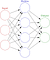Figure 1 (Source)

Figure 1 shows many circles that represent the “nodes” of a neural network. These nodes can be input nodes, output nodes or nodes that are in between input and output. They are connected by arrows which connect the nodes. This notion of connected nodes is derived from the human brain, specifically neurons which are connected in a similar manner. In essence, the working of a neural network represents neurons firing in the brain. The diagram shows three “layers” of neural networks: input, hidden and output. More complex neural networks however, have far more layers and nodes.

To represent this mathematically, the following diagram can be used.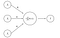Figure 2

Figure 2 represents a simple neural network focusing on a single node of the network. ‘X1’, ‘X2’, ‘X3’ represent the inputs. ‘W1’, ‘W2’, ‘W3’ are the “weights” of the neural network. A weight essentially denotes the strength or the amplitude of the connection. This is derived from the notion of a synaptic weight in the human brain. Weights are an important part of neural networks as they are altered to improve the accuracy of classification of the neural network. Most functions and algorithms in neural networks focus on adjusting the weights to make them optimal for getting the required output. The result ‘Y’ can be represented through the following equation:

## Image Classification with Neural Networks

For a computer to be able to comprehend an image, it needs to be converted to a form that a computer can understand it. There are a few ways of doing this. The image can be converted to grayscale format, which is essentially representing an image through a range of shades of grey (ranging from black to white). The computer will give each pixel a value depending on how dark it is. These values are then put into an array. Another way of doing this is through RGB (Red, Green, Blue) values. Just like in grayscale, each pixel can be assigned an RGB value that can range between 0 and 255. These values can then be put into an array. Whether, it is through grayscale or RGB, after the array has been created with many images that form the training data, the patterns can be compared to testing data and a prediction can be made.

Another method of classification is through convolution (done through convolution neural networks which is essentially a type of a neural network). The way a convolutional neural network works is by breaking the tiles into smaller pieces of tiles that can have content that overlaps. This can be represented through the following image.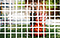Figure 3: Overlapping Image Tiles (Source)

Each of these tiles is then put into separate neural network. It is important to note that for each of the neural networks, the weights remain the same. After the processing by the neural networks is done, the results of each of the neural network is put into an array. After the arrays have been created, a process called downsampling occurs. Downsampling is essentially converting an image to a smaller scale, thus reducing its size. Downsampling is done through an algorithm called max pooling. Max pooling is used to reduce the image size while retaining the details of the image. It does so by dividing the image into 2 x 2 or even 3 x 3 matrix and choosing the highest value in those matrices. A visual representation has been given in Figure 4.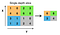Figure 4: Max Pooling (Source)

As one can infer from the working of a convolutional neural network, there are multiple steps involved in its working. When convolutional neural networks are used in real life applications which demand a high level of complexity, these steps could be repeated multiple times and in different orders as well.

# Methodology of Evaluation

This section will discuss the method which was used to evaluate the machine learning algorithm in terms of accuracy, misclassification rate and amount of training data used.

## Choosing Neural Networks

There are various reasons as to why neural networks were chosen for this investigation. The main reason is their effectiveness in image recognition and classification. This can be seen through various self-driving cars using deep learning (variation of neural networks) to detect objects in their paths. Furthermore, companies like Google use neural networks in their image based products such as Google Street View, Google Images to recognize different images. Another reason for choosing neural networks is that they can be implemented as part of supervised or unsupervised learning. In this investigation, they were implemented as supervised learning where the training data were labeled. Specifically for the experiment, a convolutional neural network was used.

## Dataset

To enable a fair evaluation, the dataset remained the same throughout the investigation. The experiment consisted of images of cats and dogs in equal amounts. The testing data was kept constant — 1000 images in total, however, the amount of training data was changed. The data for the investigation was downloaded from public datasets such as Imagenet and Cifar 100.

## Technologies Used

For this investigation, various technologies were used to conduct the experiment. The programming language used was Python 3.6. Python was chosen as it is a convenient programming language for making machine learning programs. Further, python has a lot of support in terms of libraries that can be used for machine learning. The integrated development environment that was used was IDLE. The main library used for the experiment was Keras. Keras is a high level neural network API which is capable of running on top of powerful libraries such as TensorFlow. TensorFlow is Google’s open source machine learning library which provides many tools for making machine learning programs. The reasons for choosing Keras were the following: it allows for easy and fast prototyping and supports Convolutional and Recurrent neural networks. This was crucial as, convolutional neural network was used for the experiment.

## Evaluation Criteria

The evaluation was done by calculating the accuracy and the misclassification rate of the neural network through the following formulas:

The number of Epochs was also evaluated. Epochs in machine learning can be defined as a single pass through the entire training set while training a machine learning algorithm. In a single epoch, all training samples are presented to the machine learning algorithm once.

# Findings and Evaluation

The amount of training data and testing data used for this investigation can be represented through the following table:

As one can infer from the table, the testing data was kept constant with a total of 1000 images. The training data was changed from 200 to 400 to 1000 to 2000 images. A sample of 1000 images was chosen to enable a wide variety of testing data. The training data was varied in those values to see how the classifier performs with lesser, equal to and more training data as compared to testing data. The neural network was used on the above data and the results are shown below: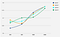Figure 5, Note: Here cycles mean epochs

Before drawing a comparison, it is to be noted that the value of each of the accuracies in each cycle is the average accuracy that is achieved in the current cycle and the previous ones. For example, in the case of training data with 200 images, the accuracy achieved in cycle 3 is the average of the accuracies achieved in Cycle 1, Cycle 2 and Cycle 3.

One would assume that Cycle 4 should do the best in all cases, however it does the worst among the other cycles in the case of training data with 1000 and 2000 images. So one can conclude that a higher amount of cycles necessarily does not mean higher accuracy. This is because there are other factors such as the dataset and the algorithm that play a part in a classifier being accurate. Further, we see that in the case of Cycle 2, the accuracy of training data with 400 images decreases as compared to training data with 200 images. As to why this happens, it will be discussed in detail in section 5.7.

In Figure 9, the highest accuracy among all the accuracies was achieved with Cycle 2 and the training data with 2000 images. The accuracy was 0.7759. Further, we can see that Cycle 1 and Cycle 3 came close to highest accuracy as well, with the same amount of training data as Cycle 2.

## Implications

Seeing Figure 5, one could also argue that overall there is only a difference of 10% in the different data points, hence why the questions of cycles and amount of training data. Looking at mathematically, it may seem there are only minor differences between the accuracies. However, when one looks at it from a real world context, one can notice the importance of those minor differences. Real world applications could be real time object detection in self driving cars or detecting skin cancer through image classification. Even a difference of 0.1% accuracy could be the difference between a car not recognizing a particular object, say a bicycle and causing road accidents. Similarly, in neural networks detecting a cancer, a minor accuracy difference could cause an image classifier not detecting cancer that a patient has. These reasons justify why there has been continual effort to research into increasing accuracy of an image classifier and the importance of even minor differences in accuracy. These seem minor mathematically, but are extremely important when put into a real world context when the actual machine learning algorithm is being applied.

## Underfitting and Overfitting

This section evaluates the drop in accuracy in Cycle 2 for the 400 images data and discusses why higher cycles or higher amount of training data can sometimes lead to lower accuracy.

A machine learning algorithm’s aim is to approximate the unknown mapping function that relates the output to the input variables. In statistics, a fit refers to how close the approximation of the current function is to the target function.

Overfitting refers to machine learning model that learns from the training data “too well”. Overfitting happens when a machine learning model learns the details and noise in the training data to the extent that it causes a negative performance of the model on new data. Noise here refers to distortion in data that is not wanted by the receiver, in this case, the machine learning algorithm. In the case of images, noise can be due to poor lighting during the capturing of the image. It can also be due to the sensor of the camera, which can be of low quality, hence not being able to capture a picture which has a high density. Any machine learning algorithm’s objective is to generalize from the training data so as to enable an accurate prediction when the testing data is used. However, if the noise is “learned” by the algorithm and becomes a concept to a model, then it will negatively impact its performance as the same noise will not be presented in the testing data. There are a few ways to deal with overfitting. One way is by using a resampling technique to estimate model accuracy or hold back a validation dataset. A popular resampling technique k-fold cross that it allows a model to train on k-times on different subsets of training data and build up an estimate of the performance on the testing data. Holding back a validation dataset essentially means taking a part of the testing data and using it later (after fine tuning the algorithm on training data) as testing data. This will give an accurate representation of how the algorithm will perform on unseen data.

Underfitting refers to a machine learning model that can neither model the training data nor generalize the new data i.e. the test data. Underfitting is easy to detect if there is a good performance metric. There is no easy solution to the underfitting problem. Sometimes it is better to try and see if there is a better machine learning algorithm that can be used. That way underfitting could be resolved.

Therefore to get optimal number of epochs and a high level accuracy from the training dataset, one can definitely use k-fold cross validation to estimate optimal number of epochs. The key here is “optimal”, which refers to the number of epochs not being neither too high nor low.

# Conclusion and Further Scope

This investigation discussed has explored how the accuracy of convolutional neural network as an image classifier using Keras API varies with the amount of training data and epochs. From the findings, one does see that there is a linear relationship between the amount of training data used and the accuracy of an image classifier in the scope of this investigation. This shows that the accuracy of an image classifier depends on the amount of training data used to a large extent. However, it cannot be concluded that there is a linear relationship between training data used and accuracy of the classifier. This is because at one point, the accuracy will become 100% and may start to decline if the training data is continually increased due to underfitting and overfitting. It is also to be kept in mind that number of epochs, dataset and algorithm also play a crucial role in determining the accuracy of an image classifier. As discussed, if the number of epochs is not optimal, it can lead to underfitting and overfitting.

The tests were conclusive, however were limited to one dataset (images of cats and dogs). Though the same classifier can be used to classify any other type of images, it was not feasible due to hardware limitations. The number of epochs that could be performed were also limited due to the same reason.

This paper examined how the amount of training data relates to the accuracy of an image classifier, specifically convolutional neural networks. To formulate more firm judgements, the algorithm could be tested in a variety of datasets and with more powerful hardware, enabling a high number of epochs and stronger comparison between the number of epochs and datasets.

Originally Written as a research paper in April 2018(Final Draft) as part of a high school research project(IBDP Extended Essay). For Bibliography & Orginal Source

## AT Blog

A Blog on technology, life, my experiences and thoughts.

## AT Blog

A Blog on technology, life, my experiences and thoughts.

Written by

## Abhinav Tripathy

Web Developer, AI Enthusiast, Student @ UMass Amherst## AT Blog

A Blog on technology, life, my experiences and thoughts.

## More From Medium

Medium is an open platform where 170 million readers come to find insightful and dynamic thinking. Here, expert and undiscovered voices alike dive into the heart of any topic and bring new ideas to the surface. Learn more

Follow the writers, publications, and topics that matter to you, and you’ll see them on your homepage and in your inbox. Explore

If you have a story to tell, knowledge to share, or a perspective to offer — welcome home. It’s easy and free to post your thinking on any topic. Write on Medium